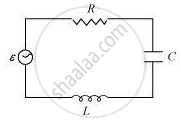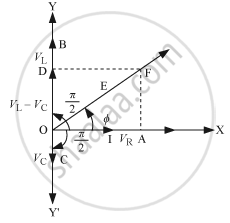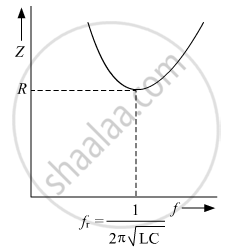# Answer the Following Question. in a Series Lcr Circuit Connected Across an Ac Source of Variable Frequency, Obtain the Expression for Its Impedance and Draw a Plot Showing Its Variation with Frequency - Physics

#### Question

In a series LCR circuit connected across an ac source of variable frequency, obtain the expression for its impedance and draw a plot showing its variation with frequency of the ac source.

#### Solution

Consider the LCR circuitAn AC source E with voltage ν = ν_m sin ωt is applied across LCR circuitAs the inductor, capacitor, and the resistor are connected in series so the current through all of them is the same (same amplitude and same phase)
Let the current be 1= Im sin wt
The voltage across each component has a different phase relation with the current.

1. Let the maximum voltage across the resistor be VR = ImR that is in the same phase of the current hence it is represented by OA in the phasor diagram.
2. Let the maximum voltage across the inductor be VL = ImXL and that leads the current by pi/2 it is represented by OD in the phasor diagram.
3. Let the maximum voltage across the capacitor be Vc = ImXc and that lags behind the current by pi/2, it is represented by OC in the phasor diagram.

Resultant voltage can be found by using the vector sum of the phasors. The resultant voltage is represented by OF.

It can be written as:

V_m = sqrt(V_R^2 + (V_L - V_c)^2)

V_m = sqrt((I_mR)^2 + (I_mX_L - I_mX_c)^2)

V_m = I_m sqrt(R^2 + (X_L - X_c)^2)

Z = V_m/I_m = sqrt(R^2 + (X_L - X_c)^2)

or, Z = V_m/I_m = sqrt(R^2 + (ωL - 1/(ωC))^2)

Variation of impedance Z with frequency f:Is there an error in this question or solution?